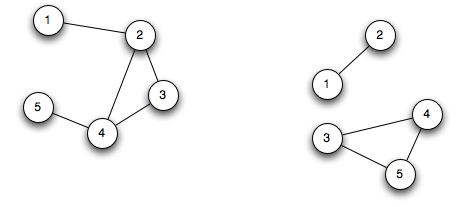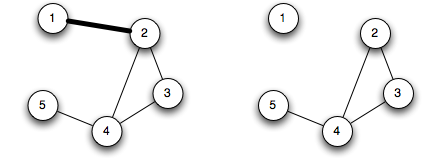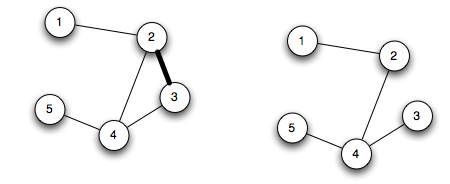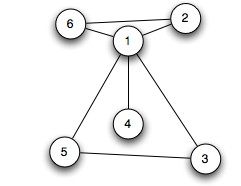# DWITE Online Computer Programming Contest

## Bridges In A Graph

November 2007
Problem 5

A graph is a collection of nodes, called vertices, connected to each other in some fashion by edges. A graph is called connected if it is possible to find a path along edges from every point to every other point.

Below are two graphs:The one on the left is connected, while the one on the right is not connected (there is no path from node 1 to node 3).

An edge of a graph is called a bridge if by removing that edge the graph is no longer connected.

Edge 1–2 in the following graph is a bridge, since by removing it, the graph is no longer connected (no path from node 1 to any other node):Edge 2–3 however, is not a bridge:You are tasked with finding the number of edges in a graph that are bridges. You will be given 5 connected graphs, and you will output 5 single integers for the number of bridges found in the graphs.

The input file is DATA5.txt. The first line will contain a single integer N, the number of vertices. 1 ≤ N ≤ 100. Vertices will be numbered from 1 to N. The second line will contain a single integer M, the number of edges. 0 ≤ M ≤ 1000. This is followed by M lines, each describing an edge. An edge is described by two integers separated by a space. All edges are valid. This format will be repeated 5 times (that is, the line after the last edge of the first graph will be a single integer describing the number of vertices in the second graph).

The output file OUT5.txt will contain 5 lines, a single integer per line – the number of bridges in the described graph.

Sample Input (only first of 5 cases shown):
```6
7
1 2
1 3
1 4
1 5
3 5
6 2
6 1```
Sample Output (only first of 5 outputs shown):
`1`

Explanation of Input:

```6 - There are 6 vertices, numbered 1 2 3 4 5 6
7 - There are 7 edges
1 2 - Vertex 1 is connected to vertex 2
1 3 - Vertex 1 is connected to vertex 3
1 4 - Vertex 1 is connected to vertex 4
1 5 - Vertex 1 is connected to vertex 5
3 5 - Vertex 3 is connected to vertex 5
6 2 - Vertex 6 is connected to vertex 2
6 1 - Vertex 6 is connected to vertex 1```

The sample input would define the following graph:The only bridge is the edge 1–4.